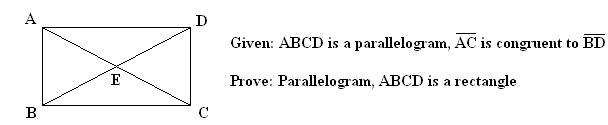Quandaries and Queries Name: An Student Level: secondary   Hi, Im taking a geometry course for the summer , and we just started to learn about proofs for about one week. Today in class, we started to do this one proof but didnt finish it because class ended. the problem is as follows.NOT DRAWN TO SCALE   This is what i have so far   Statements                                                                        Reasons 1. ABCD is a Parallelogram                                            a) given      AC is congruent to BD 2. AD is congruent to BC                                                 b) definition of parallelpgram      AB is congruent to DC 3. BD is congruent to BD                                                c) reflexive property 4. triangle ABD is conruent to triangle CDB              d) Side-Side-Side Theorem     As a class, we formulated a plan. In order to show that the parallelogram is a rectangle, we have to prove that the all the angles are 90 degreees. This is what i need help on. How do you finish this proof, and how do you prove that an angle is 90 degrees? Hi An, This is good so far. Using the same argument you can prove that triangles ABC and CDA are congruent. Then, since AC and BD are congruent you can prove that triangles ABC and ABD are congruent. Hence triangles ABC, CDA, ABD and DBC are all congruent. Can you see how to complete the proof? Penny Go to Math Central